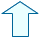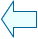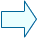LINEAR FUNCTIONS Analysis

 1. THE IDENTITY FUNCTION y = x An identity function is a function where its value on the x-axis corresponds to the same number on the y-axis. In other words, the coordinates of each of the points are the same (1,1), (2,2), (3.5,3.5). 1.- Move the red point and look carefully at how all the points on the straight line satisfy the equation y = x. 2. Change the scale to check that it is true for big and small values of x. As you can see, the graph of the identity function is a straight line, which bisects the first and third quadrants of the Cartesian coordinates system. All the points on this line have identical coordinates on the x-axis and the y-axis.

2. THE DOUBLE FUNCTION
The double function is the function where the value of the y-axis coordinate is worth double the value of the x-axis one, for example:(1,2), (3,6), (4.5,9) etc.

y = 2x

 You can move the red point with the mouse or the little arrows and also change the value of x.

3.- Look carefully at the coordinates of the double function.

4.-What do you think the graph of a double function looks like? Draw it in your exercise book.

3. COMPARING FUNCTIONS
We are going to compare the graphs of the identity and double functions to see their similarities and differences.

y = x

y = 2x

 You can move the red point with the mouse or the little arrows and you can also change the value of x.

5.- Look carefully at the graphs of the identity and double function.

6.- In your notebook write down what you think the similarities and differences are between them.

7.-What do you think the graphs of the following functions look like: triple, quadruple, quintuple, half, sixth, tenth etc?

4. LINEAR FUNCTIONS y = mx
Now, we are going to look at the graphs of some linear functions: y = 2x y = 3x y = ½ x ...
 You can see the graphs of some linear functions by using the m control button.

8.- In your exercise book draw four graphs of the following type of functions: triple, quadruple etc, and four graphs of this type of function: half, fifth etc. Find the quickest, easiest way of doing so.Juan Madrigal MugaSpanish Ministry of Education. Year 2001